All determined formulas for all the parameters of the right triangle by knowing t angle bisector and h altitude which belong to the right angle will be checked out automatically in various programs | Статья в журнале «Молодой ученый»

Отправьте статью сегодня! Журнал выйдет 18 декабря, печатный экземпляр отправим 22 декабря.

Опубликовать статью в журнале
Проекты
Меню

Рубрика: Технические науки

Дата публикации: 26.06.2018

Статья просмотрена: 2 раза

### Библиографическое описание:

Ахмаджонов, М. М. All determined formulas for all the parameters of the right triangle by knowing t angle bisector and h altitude which belong to the right angle will be checked out automatically in various programs / М. М. Ахмаджонов. — Текст : непосредственный // Молодой ученый. — 2018. — № 25 (211). — С. 107-110. — URL: https://moluch.ru/archive/211/51807/ (дата обращения: 06.12.2021).

﻿

1. Checking out the formulas in Code::Blocks program. This checking way will prove the formulas how they are correct or incorrect.

During the working in the Code::Blocks program, the special codes are inserted, F9 button will have been pressed and h altitude and t angle bisector will have been given and the values of all parameter will be shown.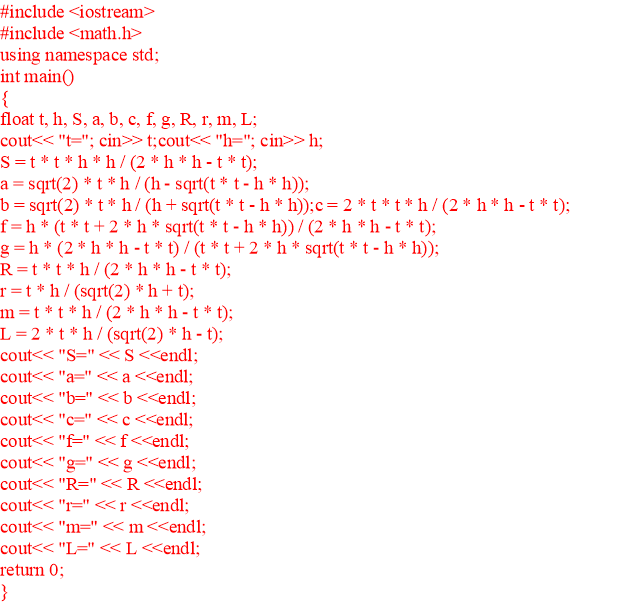The values will be inserted to t angle bisector and h altitude after the codes have been input which are stated above. For instance h = 3, t = 4are accepted in order to get all-in-one values which belong to others.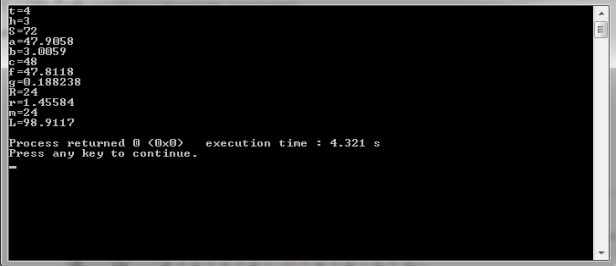1. The checking out the formulas in the Borland C++ Builder 6.

When entered to the Borland C++ Builder 6 program too, special codes are inserted, after F9 button will have been pressed and h altitude and t angle bisector of the values will have been given, «Calculate» button will be pressed and then the values of all parameters are going to be shown. The following formulas which has been needed to be inserted: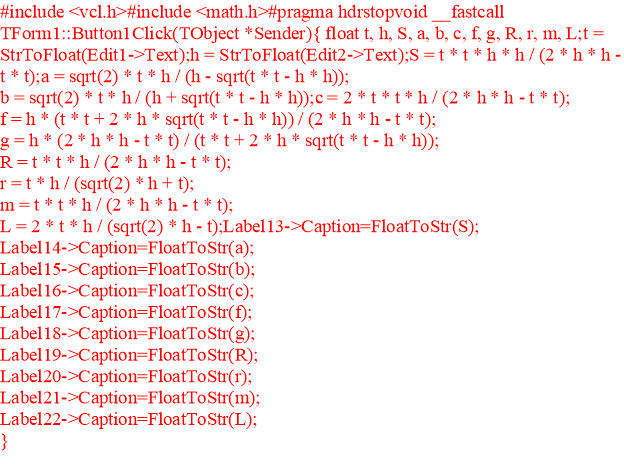The values will be inserted to t angle bisector and h altitude after the codes have been input which are stated above. For instance h = 3, t = 4 are accepted in order to get all-in-one values which belong to others. The following values of all parameters can be shown.1. The checking out the formulas in the Microsoft Excel 2010.

The specific codes will be added to the cells in this program as well. For instance, to B6 cell B6=2*B1*B1*B2/(2*B2*B2-B1*B1), namely this formula is written which determines P perimeter. After that to t and h the values is going to be input. Such as h = 3, t = 4are accepted in order to get all-in-one values which belong to others. The following values of all parameters can be viewed.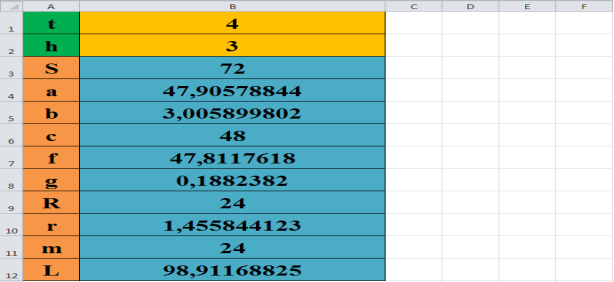№ Real results Taken results S 72 72 a 48 47.9057884216309 b 3 3.00589990615845 c 48 48

In above, the checking ways of the formulas have been seen in these three programs and all the values of the parameters have been resulted similar. That is why the respectively mistake of the formulas is calculated by comparing the real results and the taken results. Respectively mistake will be signed as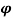.In order to calculate the respectively mistake, aleg will be taken as an example. The taken value of the a leg is a = 47.9057884216309 and the real value of the a leg should be a = 48.The following calculating the value of the respectively mistake will be shown: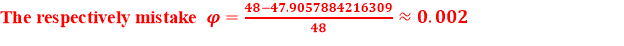In above the value of the respectively mistake of the formulas have been determined. The respectively mistake of the formulas is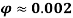.That means two percent out of thousand percent might be wrong.

References: